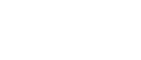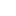#• 제품• 솔루션• 테크놀로지• 서포트• 기업정보대한민국 | Korean• Japan
• Global
• United States
• Europe
• China
• Korea
• Taiwan
• India

#• 제품
• 솔루션
• 테크놀로지
• 서포트
• 기업정보

## Semiconductor Magnetoresistive Elements(SMRE)

### Suitable for high accuracy detection of gear rotation.

Since a Sn doped single crystal film InSb is used as a material for the sensing element, the element has excellent magnetoresistive temperature characteristics and very small neutral voltage temperature drift.

This element is mainly used for detection of gear rotation.

Semiconductor magnetoresistive element MS product family comprises the back bais holder built-in dual inline package (DIP) type with A/B,Za/Zb phase output as well as small out-line package (SON) type which can be set in thin/small place with A/B phase output.

## A differential output semiconductor magnetoresistive element, (SMRE), for module, “m“ = 0.8 gear wheels

MS0083

The MS0083 is optimized for a gear wheel sized in the range from Φ60 to 100 mm.  It eliminates in-phase noise with differential output signals of A+/A- and B+/B-.  The MS0083 achieves high resolution rotation angle detection exceeding 20-bit from electronic interpolation, achieving top-level performance among magnetoresistive elements*.  This is due to its stunning temperature characteristics while having extremely small variability among individual devices.

● A+/A-, B+/B- Differential Output

● Optimized for a Specific Type of Gear

## Lineup

Semiconductor Magnetoresistive Elements

`Part Number`
```Part
Status```
```Pitch
[mm]```
```Input / Output
Resistance (MIN.)
[Ω]```
```Input / Output
Resistance (TYP.)
[Ω]```
```Input / Output
Resistance (MAX.)
[Ω]```
`Number of Elements`
`Other`
```Operating
Temperature
[℃]```
```Device
Package```
```Number
of Pins```
`MS0042`
`MP`
`1.28`
`290`

`420`
`2`

`-40 to 125`
`SON`
`8`
`MSP082`
`MP`
`2.512`
`465`

`755`
`2`

`-40 to 100`
`DIP`
`8`
`MS0041`
`MP`
`1.28`
`270`

`375`
`1`

`-40 to 125`
`SON`
`4`
`MSP043`
`MP`
`1.28`
`230`

`375`
`2`

`-40 to 100`
`DIP`
`8`
`MS0083`
`MP`
`2.624`
`290`

`480`
`1`

`-40 to 125`
`SON`
`6`
`MSP204`
`MP`
`6.84`
`290`

`420`
`4`

`-40 to 125`
`DIP`
`8`
`MS0020`
`MP`
`0.634`
`205`

`330`
`1`

`-40 to 100`
`SON`
`4`
`MS0050`
`MP`
`1.6`
`270`

`375`
`1`

`-40 to 125`
`SON`
`4`
`MS0043`
`MP`
`1.28`
`275`

`410`
`1`

`-40 to 125`
`SON`
`6`
`MS0030`
`MP`
`0.96`
`270`

`375`
`1`

`-40 to 125`
`SON`
`4`
`MS0082`
`MP`
`2.512`
`465`

`755`
`1`

`-40 to 125`
`SON`
`4`
`MSP042`
`MP`
`1.28`
`230`

`375`
`2`

`-40 to 100`
`DIP`
`8`
`MSP254`
`MP`
`8.64`
`290`

`420`
`4`

`-40 to 125`
`DIP`
`8`